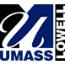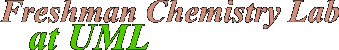Experiment

1

Weighing

## Overview

The ability to read the numerical scale of a measuring device will be very important to you in your study of chemistry and other sciences. This experiment, although framed in terms of determining the mass of some small "unknown" objects, is really an exercise in significant figures and how to read an analog scale correctly, to the limits of precision permitted by the scale. Generally, we read the scale of any measuring device to one-tenth (0.1) unit of the smallest marked scale division.

## Calculations

### Report Page 5

Suppose you had determined the follow three values for the mass of the centigram unknown:

 Trial 1 Trial 2 Trial 3 10.143 g 10.115 g 10.124 g

The mean mass of the unknown is then the sum of the individual measurements, divided by the number of measurements (i.e., three). Calculate the mean mass of the three measurements of mass above. Then click here to see if you did the calculation correctly.

The deviations of the individual results from the mean result are then calculated as follows: subtract the mean from each of the individual masses determined. Using the individual masses for the three trials above, and the mean mass you calculated, calculate the deviations from the mean for each trial. Then click here to see if you did the calculation correctly.

The average deviation, is then the sum of the absolute values of the individual deviations, divided by the number of measurements (three). For the three individual deviations you calculated above, calculate the average deviation. Then click here to see if you did the calculation correctly.

Another means of expressing the precision of a measurement is in terms of the percent deviation. For the data above, the percent deviation would be given bySuch a small percent deviation shows that the three measurements of mass were relatively consistent with one another.

If you are still having trouble with this report after having worked through and studied the examples on these pages, please see a tutor at the Freshman Lab Help Center (OH223b) as soon as possible.